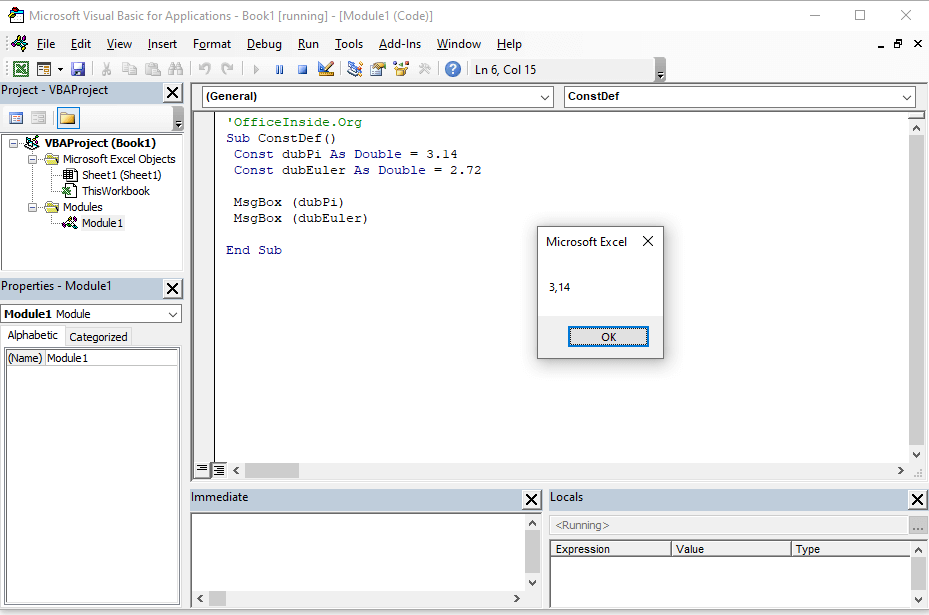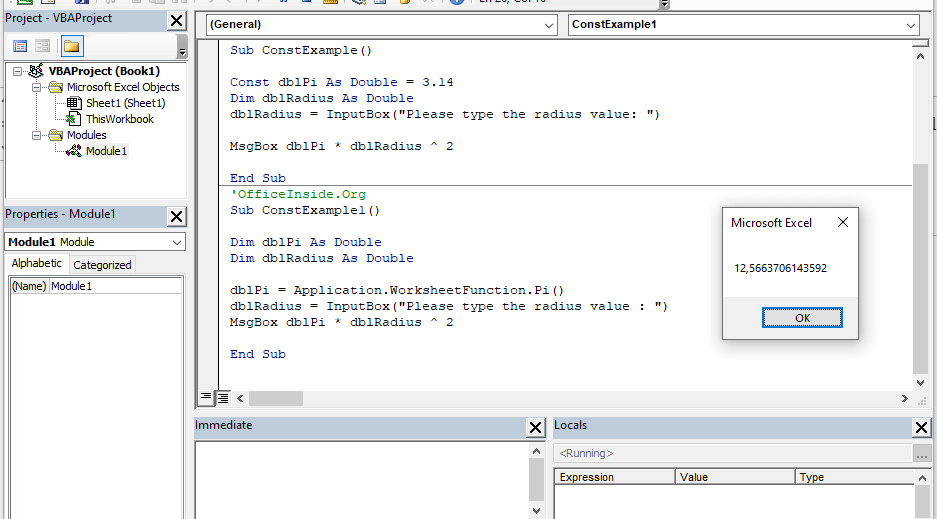# Constant values in VBA Excel programming

## Constant values in VBA – what is it?

In the previous chapter of the course you learned what are the VBA variables using in your VBA code. The difference between variables and constants in VBA is the values ​​assigned as constant will not change during the launch of your program. If you want your code to be fully compliant with the principles of good programming you should use a separate declaration type.

You can use the definition of  Const . You must assign the value for the constant immediately after declaring it. It will not be possible to change it in next blocks of code. Below is an example of declaring the number Pi and the Euler number as constant in VBA. Importantly, the data type has been specified. However, it is not required when declaring VBA constants.

```'OfficeInside.Org
Sub ConstDef()
Const dubPi As Double = 3.14
Const dubEuler As Double = 2.72

MsgBox (dubPi)
MsgBox (dubEuler)

End Sub```Constant values in VBA – what is it?

## Example: How to use constant values in VBA?

Let’s create a short program that, after entering the radius of a circle, will calculate its field. Apply the InputBox and MsgBox windows for this purpose.

```'OfficeInside.Org
Sub ConstExample()

Const dblPi As Double = 3.14
Dim dblRadius As Double
dblRadius = InputBox("Please type the radius value: ")

MsgBox dblPi * dblRadius ^ 2

End Sub```

In this example, we set the number of PI value in the VBA code, assigning it a constant value 3.14. This number can also be entered into the program using the Excel spreadsheet function. To do this, you can use the Application.WorksheetFunction.NAME_FUNCTION  command that allows you to use the excel spreadsheet functions.

```'OfficeInside.Org
Sub ConstExample1()

Dim dblPi As Double
Dim dblRadius As Double

dblPi = Application.WorksheetFunction.Pi()
dblRadius = InputBox("Please type the radius value : ")
MsgBox dblPi * dblRadius ^ 2

End Sub```Example: How to use constant values in VBA?

## Tasks (You can enter the solution in a comment)

1. Using the Range or Cells command, modify the example and calculate the circle field by taking the radius value from the cells of the sheet. The result itself should also appear in the sheet.
2. Using constants, create a program based on the InputBox and MsgBox windows and calculate the circumference of a circle with radius 10. As a reminder, the pattern on the circumference of a circle is. = 2 * Pi * R

More examples of Excel VBA basics can be found in the Excel VBA Course section and  Excel VBA How To section. If you have any questions about this chapter, you can post them in .